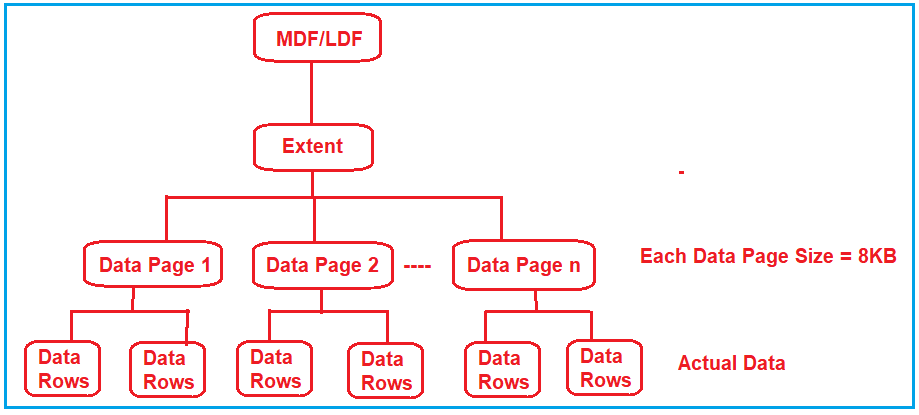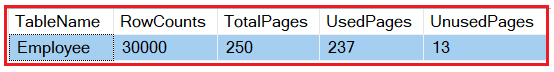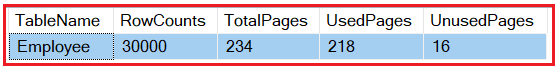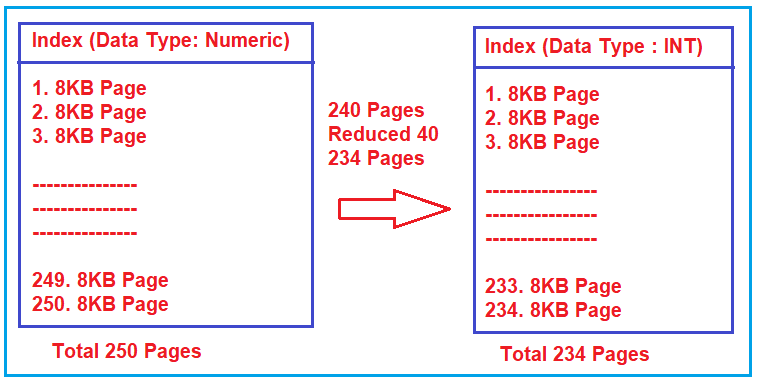# Create Index on Proper Column to Improve Performance

## Create Index on Proper Column to Improve Performance in SQL Server

In this article, I am going to discuss how to Create Index on Proper Column to Improve Performance in SQL Server. Please read our previous article where we discussed how to use Covering Index to reduce RID lookup which will improve the SQL query performance.

Note: Keep the index size as small as possible.

Let me explain how to choose the proper column for creating an index in order to improve the performance of your SQL query. Suppose you have two columns and you have to choose the column for creating an index. One column data type is int whereas the other column data type is numeric. So, here you should choose the int data type column for creating index rather than the numeric data type. And the reason for this is, int data type size is small as compared to the numeric data type size.

##### How the index size increase and decreases the SQL Query performance?

In order to understand this, first, we need to understand how the data are physically stored and how the data pages are worked. Please have a look at the following diagram which shows the internal architecture of how the data are physically stored in SQL Server.At the top you can see the MDF / LDF files; these are actually the physical files that exist on your machine. These MDF/LDF files are further divided into something called Extent. Extent is nothing but the logical grouping of pages which you can see in the above image. Inside the Pages or Data Pages, you have the actual data rows. So, you can think the pages are the fundamental unit of storage. Each data page size is approximately 8KB. Suppose, you have a table with 50 rows, then it is possible that it store the 25 rows in Data Page 1 and the next 25 rows in the 2nd Data Page depending on the size of a row.

Note: The more the number of data pages, the SQL Server engine has to take more time to get the data as it has to jump between multiple pages. On the other hand, the less the number of data pages, the SQL Server engine takes less time to get the data as there is less number of jumps between the data pages.

Let say you have a table having 100 rows and the total size of all the 100 rows is less than 8KB. So, they all are going to be stored on a single data page. So, here the SQL Server engine can quickly go to that page and quickly browses that record and it does not have to jump between the pages.

Let say the same 100 records are pushed into four data pages. Now, the SQL Server engine has to jump between these four pages to get the records. And this jump between the pages takes time.

So, let see practically when we create an index on int data type how the page sizes are less as compared to when we create an index on the numeric or decimal data type.

##### Example to show how the index size increase and decrease the performance:

Let us see an example to understand how the index size increases and decreases the performance of SQL Server. Here, we are going to create the following Employee table. This table at the moment does not have any keys or indexes. Please execute the following SQL Script to create the Employee table.

```CREATE TABLE [Employee](
[EmployeeID] INT NOT NULL,
[EmployeeName] VARCHAR(50),
[EmployeeCode] NUMERIC(18, 0) NOT NULL
)
GO
```

Now let us insert a huge amount of records by using a loop. So, please execute the following to insert 30000 records into the Employee table.

```DECLARE @CODE NUMERIC(18, 0), @ID INT;
DECLARE @Name VARCHAR(20)
SET @CODE = 10000;
SET @ID = 1;
WHILE @CODE < 40000
BEGIN
SET @Name = 'Name - ' + CAST(@ID AS VARCHAR(10))
INSERT INTO Employee VALUES(@ID, @Name, @CODE)
SET @ID = @ID + 1
SET @CODE = @CODE + 1
END;
```
##### Scenario 1: Creating Index on Numeric Datatype column

Let us first create one index on the EmployeeCode column whose data type is Numeric. So, please execute the following script to create the index.

CREATE NONCLUSTERED INDEX IX_Employee_CODE ON Employee (EmployeeCode);

Once you created the index on the EmployeeCode column, then in order to see the number of data pages along with the used and unused data pages please execute the following script. Explaining the following script is beyond this article and I have taken this script from stack overflow.

```SELECT
t.NAME AS TableName,
p.rows AS RowCounts,
SUM(a.total_pages) AS TotalPages,
SUM(a.used_pages) AS UsedPages,
(SUM(a.total_pages) - SUM(a.used_pages)) AS UnusedPages
FROM
sys.tables t
INNER JOIN
sys.indexes i ON t.OBJECT_ID = i.object_id
INNER JOIN
sys.partitions p ON i.object_id = p.OBJECT_ID AND i.index_id = p.index_id
INNER JOIN
sys.allocation_units a ON p.partition_id = a.container_id
WHERE
t.NAME = 'Employee'
AND t.is_ms_shipped = 0
AND i.OBJECT_ID > 255
GROUP BY
t.Name, p.Rows
ORDER BY
t.Name
```

When you execute the above query, it will give you the following output.As you can see, the total number of pages is 161.

##### Scenario 2: Creating Index on Int Datatype column

Now, delete the clustered index and again create the clustered index on the int data type column i.e. on the EmployeeID column and then we will see the total number of data pages.

DROP INDEX Employee.IX_Employee_CODE;
GO
CREATE NONCLUSTERED INDEX IX_Employee_ID ON Employee (EmployeeID);

Now execute the following query to get the total number of pages created by this index.

```SELECT
t.NAME AS TableName,
p.rows AS RowCounts,
SUM(a.total_pages) AS TotalPages,
SUM(a.used_pages) AS UsedPages,
(SUM(a.total_pages) - SUM(a.used_pages)) AS UnusedPages
FROM
sys.tables t
INNER JOIN
sys.indexes i ON t.OBJECT_ID = i.object_id
INNER JOIN
sys.partitions p ON i.object_id = p.OBJECT_ID AND i.index_id = p.index_id
INNER JOIN
sys.allocation_units a ON p.partition_id = a.container_id
WHERE
t.NAME = 'Employee'
AND t.is_ms_shipped = 0
AND i.OBJECT_ID > 255
GROUP BY
t.Name, p.Rows
ORDER BY
t.Name
```

When you execute the above query, it will give you the below output.Now, you can see, with the int data type column index it creates a total of 234 pages to store the data. On the other hand, with the Numeric data type column index, it creates a total of 250 pages to store the same records.So, whenever you have given a chance to create an index between two data types, whichever data type is having less size should be chosen as the index (either clustered or non-clustered).

That’s it for today. In the next article, I am going to discuss how to improve the SQL Server Performance using Database Engine Tuning Advisor and SQL Profiler in detail. Here, in this article, I try to explain how to create an Index on Proper Column to Improve Performance in SQL Server in detail.

### 1 thought on “Create Index on Proper Column to Improve Performance”

1.Can you please modify in this mentioned line, where total number of pages is 250 as per your query result.

As you can see, the total number of pages is 161.